A New Energy Efficient Clustering-based Protocol for Heterogeneous Wireless Sensor Networks | OMICS International
Journal of Electrical & Electronic Systems

# A New Energy Efficient Clustering-based Protocol for Heterogeneous Wireless Sensor Networks

Nuray AT* and Daraghma SM

Department of Electrical and Electronics Engineering, Anadolu University, Turkey

*Corresponding Author:
Nuray AT
Department of Electrical and Electronics Engineering
Tel: +90 222 335 0580
E-mail: [email protected]

Received Date: August 13, 2015; Accepted Date: September 21, 2015; Published Date: October 25, 2015

Citation: Nuray AT, Daraghma SM (2015) A New Energy Efficient Clusteringbased Protocol for Heterogeneous Wireless Sensor Networks. J Electr Electron Syst 4:161. doi:10.4172/2332-0796.1000161

Copyright: © 2015 Nuray AT, et al. This is an open-access article distributed under the terms of the Creative Commons Attribution License, which permits unrestricted use, distribution, and reproduction in any medium, provided the original author and source are credited.

Visit for more related articles at Journal of Electrical & Electronic Systems

#### Abstract

Energy efficiency is one of the most important design goals for wireless sensor networks (WSNs). To this effect, clustering is mostly used to prolong the lifetime of WSNs. In this study, we propose a new energy efficient (EE) clusteringbased protocol for single-hop, heterogeneous WSNs. The proposed protocol uses channel state information (CSI) in the selection process of Cluster Heads (CHs). It is shown through simulations in MATLAB that the proposed protocol has 1.62 to 1.89 times better stability period than that of a well-known protocols including LEACH, DEEC, and SEP.

#### Keywords

Wireless sensor network, Sensor node, Clustering, Routing protocols, Residual energy, Energy efficient routing, Network lifetime

#### Introduction

Sensor networks can contain hundreds to thousands sensing nodes. It is desirable to make these nodes as cheap and energy-efficient as possible and rely on their large numbers to obtain high quality results. Wireless Sensor Networks (WSNs) are used for a variety of tasks including detection, localization and tracking objects of interest. In , an accurate estimate of a source location is obtained by using energy readings of sensors.

One challenge faced by WSNs is the power management issue. As sensors have limited energy, it needs to be used wisely so that a WSN has a long lifetime. Clustering [2-4] is one of the successful techniques to improve the lifetime of a WSN. In this approach, a network field is divided into sub regions, called clusters. Each cluster has a Cluster Head (CH) which is responsible for collecting data from member nodes within its cluster and transmitting the data to the Base Station (BS). However, this technique comes with its own problems such as deciding the number of clusters and CH to member ratio, selection and rotation process of CH(s).

Most of the analytical results in the literature assume that all sensor nodes in the network have equal energy. These networks are called homogeneous networks. In some cases on the other hand, some nodes in the network have different energies. For example, as the lifetime of a sensor network is limited there is a need to re-energize the network by adding more nodes. These nodes will be equipped with more energy than nodes that are already in use which will create heterogeneity in the network resulting heterogeneous networks .

The communications between entities on a network are governed by protocols. Network protocols must be designed to achieve fault tolerance in the presence of individual node failure(s) and to minimize energy consumption. Moreover, since the limited wireless channel bandwidth is shared among all sensors in the network, routing protocols should be able to perform a local collaboration to reduce bandwidth requirements.

A protocol called LEACH (Low-Energy Adaptive Clustering Hierarchy) was developed in [6,7]. It is a clustering-based protocol that minimizes energy dissipation in a sensor network. It is shown that LEACH outperforms classical clustering algorithms since it uses adaptive clusters, rotates CHs, and allows energy requirements of the system to be distributed among all sensors in the network. But then, when aCH dies in LEACH that cluster will become useless since data gathered at the CH will never reach to the base station.

In this study, we assume that the BS is not energy limited, the dimensions of the field and the coordinates of the BS are known. We propose a new clustering-based energy-efficient (EE) protocol for single-hop, heterogeneous WSNs. In EE-Heterogeneous LEACH, CHs are selected by using weighted probabilities. These weighted probabilities are evaluated based on the ratio between residual energy and the best channel of each node and average energy of the network. The rotating epoch (time interval) for each node is different according to its initial and residual energy. Nodes with high initial and residual energy will be more likely to become CHs per round per epoch. CHs collect data from member nodes in their respective clusters, aggregate the received data and send it to the BS using single-hop communication. Simulation results show that the proposed protocol extends network lifetime and improves energy consumption compared to other wellknown protocols including LEACH, DEEC, and SEP.

The outline of the paper is as follows. In Section 2, the previous work on the subject is summarized. The proposed protocol is presented in Section 3 and its performance is evaluated through simulations via MATLAB in Section 4. Some concluding remarks are given in Section 5.

#### System Model

In this study, we use a radio energy dissipation model given in Figure 1. Here, L bit data packets are transmitted to a receiver (Rx) located at a distance d from the transmitter (Tx). Eelec is the amount of energy needed in Tx or Rx hardware to send or receive data. Due to path loss and multipath fading phenomena that occur in wireless channels, Tx is equipped with an amplifier. The amplifier has a gain of where denotes the path loss exponent. Note that the value of the path loss exponent is between 2 and 4 in general.

To transmit L-bit message to a distance d: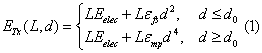where is the amplifier energy per bit per square meter (m2) when free space model is used for the channel and is the amplifier energy per bit per m4 when multipath propagation model is used. The threshold distance d0 in (1) is given by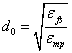and is set to 87 m in this study. Similarly, at the Rx side: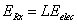(2)

Since transmitting a message is a costly operation in wireless channels, protocols used in WSNs should try to minimize not only the transmit distances but also the number of transmit and receive operations for each message.

Optimal clustering

We assume that N nodes are uniformly distributed over a square field (D x D). The square field has an area of D2 square meters and the BS is located at the center of the field for simplicity. The field is divided into Ksub regions, clusters. For each cluster, one node is assigned as the cluster head. During transmission, each non-cluster head (non- CH) node sends L bit data to the CH node within its cluster. Thus, the energy used by a non-CH node is :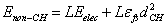(3)

where is the average distance between a cluster member and its corresponding CH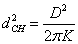Similarly, the energy dissipated in aCH is given by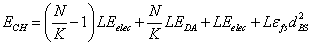(4)

where is the data aggregation processing cost and is the average distance between a CH and the BS given by :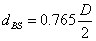The total energy dissipated during one round is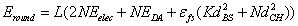(5)

By differentiating with respect to and equating to zero, the optimal number of clusters is found to be [16,17]: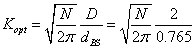(6)

If a significant percentage of nodes are farther away from the BS (a distance greater than d0), then the optimal number of clusters is given by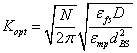(7)

The optimal probability of a node being selected as a CH is computed as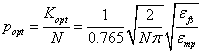(8)

The quantity plays an important role in the operations of WSNs. If the clusters are not constructed in an optimal way, the total energy consumed during a round will increase in a nonlinear fashion [17,18].

Proposed protocol

Like LEACH, our proposed protocol also consists of two phases (1) Setup Phase and (2) Steady Phase.

During the Setup Phase, the BS broadcasts a message at a certain power containing its identification information. This message makes each node aware of the BS. The BS decides the number of and optimal size of clusters depending upon the size of a network area and density of nodes. The BS then sends control packets to each node informing about the protocol to be used. These control packets consist of all necessary information required for steady state working of the protocol including threshold energy value for a CH change, TDMA slots for intra-cluster communication, CDMA code for communication with the BS along with node identities. This makes each node aware of the other members of its cluster, current round CH, CH rotation sequence, sleep and wakeup patterns to reduce collision and to save energy. Threshold energy is the least amount of energy to have for a CH node .

During the Steady Phase, non-CH nodes collect data and send to its CH (inter-cluster communication). Similarly, each CH sends data to the BS (intra-cluster communication). After each round, a CH checks its remaining energy to see if it is reached to the threshold value (Eth) for a CH change. If so, it generates a beep signal and lets its cluster members know a CH is about to change. Thereafter, it behaves like a non-CH node, i.e., it collects data and sends to the new CH. The process of CH rotation continues till each node within the cluster gets a chance to be a CH. After the last node is reached to the threshold value, the algorithm starts over and runs the same CH schedule.

In short,

1. The BS is fixed (not mobile) and located far from sensor nodes.

2. All sensor nodes in the network are uniformly distributed over a square field.

3. Sensor nodes and the BS are left unattended after their deployment.

4. A network consists of heterogeneous nodes in terms of energy.

5. All sensor nodes are of equal significances.

6. The BS has a rechargeable battery.

LEACH is an iterative algorithm and each iteration in the algorithm is called a ‘round’. The round r is referred to a time interval where all cluster members transmit to the CH. In LEACH, during the setup phase, each node generates a random number between 0 and 1. If this random number is less than a specific value, the threshold value T(s), then the node becomes a CH for that round. The threshold value is chosen as: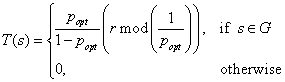(10)

where is the desired percentage of CHs among all nodes, r is the current round number, G is a set of sensor nodes that have not been selected as CHs in the last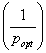rounds, and s is the current CH node.

Most energy-efficient schemes in the literature aim at minimizing the average wasted energy. To this affect, they only take into account the residual energy. On the other hand, other energy-efficient schemes focus on minimizing the average transmission energy and take into account the CSI. It is a well-known fact that network lifetime depends on both the average wasted energy and the average transmission energy Since the ultimate goal is to maximize the network lifetime, one needs to balance the both schemes. In this study, we consider the case where the CSI is available .

The received signal at the BS due to the CH is: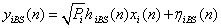(11)

where is the transmission power of the CH node i, is the channel gain between the CH node i and the BS (circularly-symmetric Gaussian random variable with zero mean and variance), and is the M-PSK modulated transmitted signal (M=2k with k positive integer) with unit average power, and is an additive noise term, circularly-symmetric Gaussian random variable with zero mean. The variance of is given by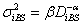(12)

where is the distance between CH node i and the BS, is the path loss exponent, and is a constant whose value depends on the propagation environment .

We propose a new threshold value T(s), an improvement to (10), as follows: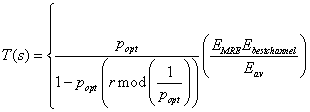(13)

where denotes the maximum of minimum residual energies, is the largest variance (or, energy) of channel gains , and is the average energy of the network.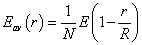(14)

where R is the network lifetime, which assumes that every node consumes the same amount of energy in each round. Suppose also that all nodes die at the same time; hence, R is the total number of rounds in which the network is alive. Let denote the energy consumed by the WSN in each round. Then R is given by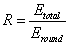(15)

In this study, we consider a WSN that uses a singlehop communication. An illustrative example of a single-hop communication with four clusters is depicted in Figure 2 where each non-CH node communicates directly (single-hop) with its CH and each CH communicates directly with the BS.

The data retrieval operation is initiated by the BS which broadcasts the request to send (RTS) message to activate sensors. CHs are enabled and data packets are transmitted to the BS through the wireless channel. All sensor nodes that have received RTS will contend for the data channel according to the non-cooperative schemes presented in . At any time instant, only one CH occupies the channel to transmit a data packet to the BS. When one transmission is being held, other CH nodes in the network are in a sleeping state.

The average BER performance for a non-cooperative node with M-PSK modulation is upper-bounded by :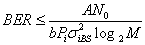(16)

where N0 is the power spectral density of the additive noise term , A and b are defined as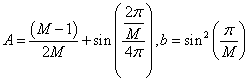(17)

If the average BER is required to be less than or equal to a given value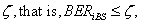the minimum transmission power is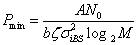Thus,where Pmax denotes the maximum transmission power.

#### Simulation Results

The performance of the proposed clustering-based protocol is evaluated using MATLAB both for homogeneous and heterogeneous networks. In the network, 100 nodes are randomly deployed in a 100 m × 100 m region where the BS is located at the center as illustrated in Figure 3.

The performance evaluation of the proposed network is done with respect to the following parameters:

Stability period: The time interval between the start of the network operation and the death of the first sensor node also known as stable region.

Instability period: The time interval between the death of the first sensor node and of the last sensor node.

Network lifetime: The time interval between the start of the network operation and the death of the last sensor node.

Number of alive nodes: The number of sensor nodes that have not yet depleted their energy.

Number of dead nodes: The number of sensor nodes that have consumed all of their energy and are not able to do any kind of functionality [22-24].

Throughput: The rate of data sent from cluster heads to the base station.

The proposed algorithm is compared with LEACH, DEEC, and SEP in terms of dead and alive nodes per round, energy consumption of the network, and overall through put. The total number of rounds used in our experiments is 8000.

Network model parameters are summarized in Table 1.

Parameter name Value
E0 0.5 J
Packet size 4000 bits
Eelec 50 nJ/bit
Etx  = Erx 50 nJ/bit
Efs 10 pJ/bits/m2
Emp 0.0013 pJ/bits/m2
EDA 5 nJ
Fc 1 GH
N0 -40 dBm
BER 10−3
η 1
α 2
Pmax 0.2
Data rate R 104
Modulation type BPSK
Ec 2x10−4
Ecs 2x10−4
Ees 10−4

Table 1: Network model parameters.

Figure 4 shows the number of dead nodes per round indicating stability time of the networks. The death of the first node occurs at the round 1270 in the proposed protocol whereas the death of the first node occurs at rounds 984, 1140, and 912 in LEACH, DEEC, and SEP, respectively. The death of the last node occurs at the round 2529 in the proposed protocol whereas the death of the last node occurs at rounds 1450, 1554, and 2115 in LEACH, DEEC and SEP, respectively. Hence, the proposed protocol has better stability time and network lifetime as compared to the other networks.

The proposed protocol has also better energy consumption and higher throughput than the other protocols considered which can be seen from the Figures 5 and 6. Initial energy E0 of the network is consumed at the round 2100 in the proposed protocol whereas the initial energy E0 of the network is consumed at rounds 1100, 1200 and 1300 in LEACH, DEEC and SEP, respectively (Figure 5). Similarly, Figure 6 shows the superiority of the proposed algorithm in terms of the throughput thanks to the wiser selection of CHs .

Here all nodes have different amount of initial energies. The initial energies are uniformly distributed on [0.5, 1] resulting the average initial energy of 0.75 J. Several experiments are conducted, the average stability periods are calculated, and the results are shown in Figures 7-9. The results show that on the average the proposed algorithm has 1.62 to 1.89 times better stability period compared to other protocols considered. Figure 7 shows the number of dead nodes per round indicating stability time of the networks. The death of the first node occurs at the round 1894 in the proposed protocol whereas the death of the first node occurs at rounds 1036, 1322, and 1243 in LEACH, DEEC, and SEP, respectively. Hence, the proposed protocol has better stability time and network lifetime as compared to the other networks. The proposed protocol has also better energy consumption and higher throughput than the other protocols considered which can be seen from the Figures 8 and 9.

#### Conclusion

This paper presents a new clustering-based protocol, EEHeterogeneous LEACH, for WSNs. In the proposed protocol, nodes with different energy levels are considered causing heterogeneity in the network. Moreover, a single-hop transmission approach is adopted for intra-cluster and inter-cluster communication. We proposed an optimized routing scheme where the main focus is to enhance cluster head selection process. CHs are selected in each cluster on the basis of residual node energy and the best channel.

From the conducted experiments, it is seen that:

Stability period of the network is enhanced compared to the other protocols LEACH, DEEC, and SEP. Superior network lifetime is obtained for different scenarios. Last but not least, the throughput of the proposed protocol is significantly better than the other protocols considered. Thus, we claim that the proposed protocol has improved energy efficiency and effective in prolonging the network lifetime.

#### References

Select your language of interest to view the total content in your interested language

### Article Usage

• Total views: 10473
• [From(publication date):
December-2015 - Jan 23, 2020]
• Breakdown by view type
• HTML page views : 10072Can't read the image? click here to refresh